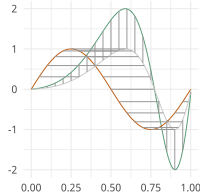# Curve Registration¶

statistic calculation for SRVF (curves) open and closed using Karcher Mean and Variance

moduleauthor:: J. Derek Tucker <jdtuck@sandia.gov>

class curve_stats.fdacurve(beta, mode='O', N=200, scale=False)[source]

This class provides alignment methods for open and closed curves using the SRVF framework

Usage: obj = fdacurve(beta, mode, N, scale) :param beta: numpy ndarray of shape (n, M, N) describing N curves in R^M :param mode: Open (‘O’) or closed curve (‘C’) (default ‘O’) :param N: resample curve to N points :param scale: scale curve to length 1 (true/false) :param q: (n,T,K) matrix defining n dimensional srvf on T samples with K srvfs :param betan: aligned curves :param qn: aligned srvfs :param basis: calculated basis :param beta_mean: karcher mean curve :param q_mean: karcher mean srvf :param gams: warping functions :param v: shooting vectors :param C: karcher covariance :param s: pca singular values :param U: pca singular vectors :param coef: pca coefficients :param pca principal directions :param qun: cost function :param samples: random samples :param gamr: random warping functions :param cent: center :param scale: scale :param len: length of curve :param len_q: length of srvf :param mean_scale mean length :param mean_scale_q mean length srvf :param E: energy

Author : J. D. Tucker (JDT) <jdtuck AT sandia.gov> Date : 26-Aug-2020

karcher_cov()[source]

This calculates the mean of a set of curves

karcher_mean(rotation=True, parallel=False, cores=-1, method='DP')[source]

This calculates the mean of a set of curves :param rotation: compute optimal rotation (default = T) :param parallel: run in parallel (default = F) :param cores: number of cores for parallel (default = -1 (all)) :param method: method to apply optimization (default=”DP”) options are “DP” or “RBFGS”

plot()[source]

plot curve mean results

sample_shapes(no=3, numSamp=10)[source]

Computes sample shapes from mean and covariance

Parameters: no – number of direction (default 3) numSamp – number of samples (default 10)
shape_pca(no=10)[source]

Computes principal direction of variation specified by no. N is Number of shapes away from mean. Creates 2*N+1 shape sequence

Parameters: no – number of direction (default 3)
srvf_align(rotation=True, parallel=False, cores=-1, method='DP')[source]

This aligns a set of curves to the mean and computes mean if not computed :param rotation: compute optimal rotation (default = T) :param parallel: run in parallel (default = F) :param cores: number of cores for parallel (default = -1 (all)) :param method: method to apply optimization (default=”DP”) options are “DP” or “RBFGS”

curve_stats.randn(d0, d1, ..., dn)

Return a sample (or samples) from the “standard normal” distribution.

Note

This is a convenience function for users porting code from Matlab, and wraps standard_normal. That function takes a tuple to specify the size of the output, which is consistent with other NumPy functions like numpy.zeros and numpy.ones.

Note

New code should use the standard_normal method of a default_rng() instance instead; please see the random-quick-start.

If positive int_like arguments are provided, randn generates an array of shape (d0, d1, ..., dn), filled with random floats sampled from a univariate “normal” (Gaussian) distribution of mean 0 and variance 1. A single float randomly sampled from the distribution is returned if no argument is provided.

d0, d1, …, dn : int, optional
The dimensions of the returned array, must be non-negative. If no argument is given a single Python float is returned.
Z : ndarray or float
A (d0, d1, ..., dn)-shaped array of floating-point samples from the standard normal distribution, or a single such float if no parameters were supplied.

standard_normal : Similar, but takes a tuple as its argument. normal : Also accepts mu and sigma arguments. Generator.standard_normal: which should be used for new code.

For random samples from $$N(\mu, \sigma^2)$$, use:

sigma * np.random.randn(...) + mu

>>> np.random.randn()
2.1923875335537315  # random


Two-by-four array of samples from N(3, 6.25):

>>> 3 + 2.5 * np.random.randn(2, 4)
array([[-4.49401501,  4.00950034, -1.81814867,  7.29718677],   # random
[ 0.39924804,  4.68456316,  4.99394529,  4.84057254]])  # random